Function Repository Resource:

# RicciScalar

Compute the Ricci scalar for a metric

Contributed by: Wolfram Staff (original content by Alfred Gray)
 ResourceFunction["RicciScalar"][M,{u,v}] computes the Ricci scalar for a metric M in terms of variables u and v.

## Details and Options

The scalar curvature is the contraction of the Ricci tensor, and is written as R without subscripts or arguments: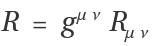.
The Ricci scalar curvature has a meaning very similar to the Gaussian curvature.

## Examples

### Basic Examples (2)

 In:=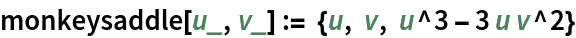In:=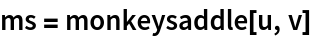Out=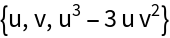Plot the surface:

 In:=Out=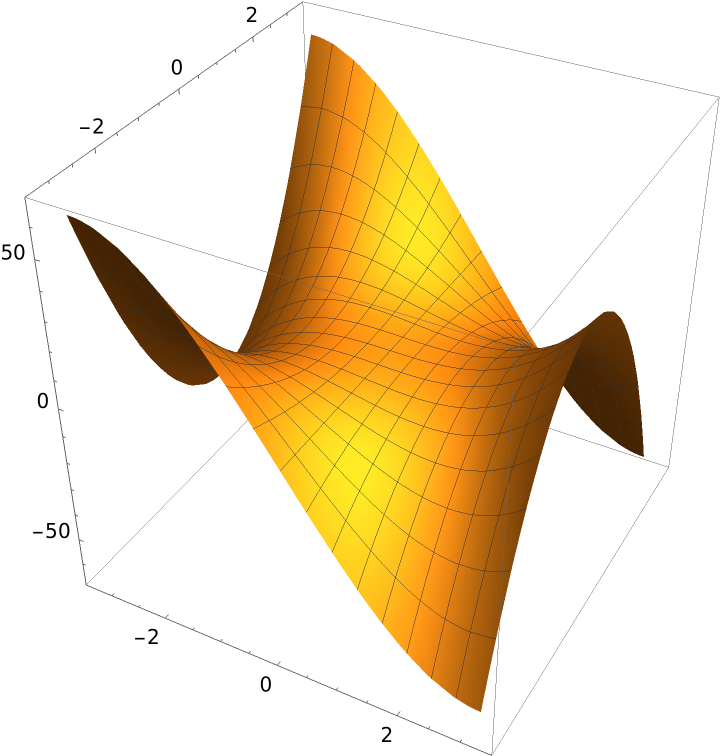The covariant basis:

 In:=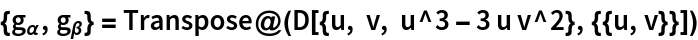Out=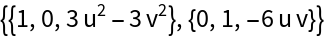The metric tensor:

 In:=Out=The metric tensor in normal form:

 In:=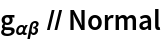Out=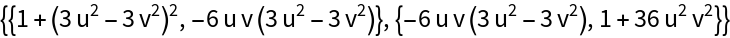Compute the Ricci scalar from the metric:

 In:=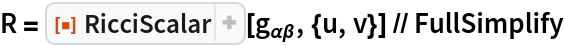Out=Alternately, compute the Ricci scalar via the Ricci curvature:

 In:=Out=Contracting an index gives the Ricci scalar:

 In:=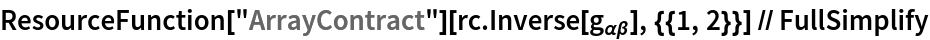Out=Confirm that this is equivalent to the Ricci curvature computed previously from the metric directly:

 In:=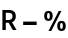Out=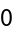The metric tensor for an arbitrary metric:

 In:=Out=The Brioschi formula for the Gaussian curvature:

 In:=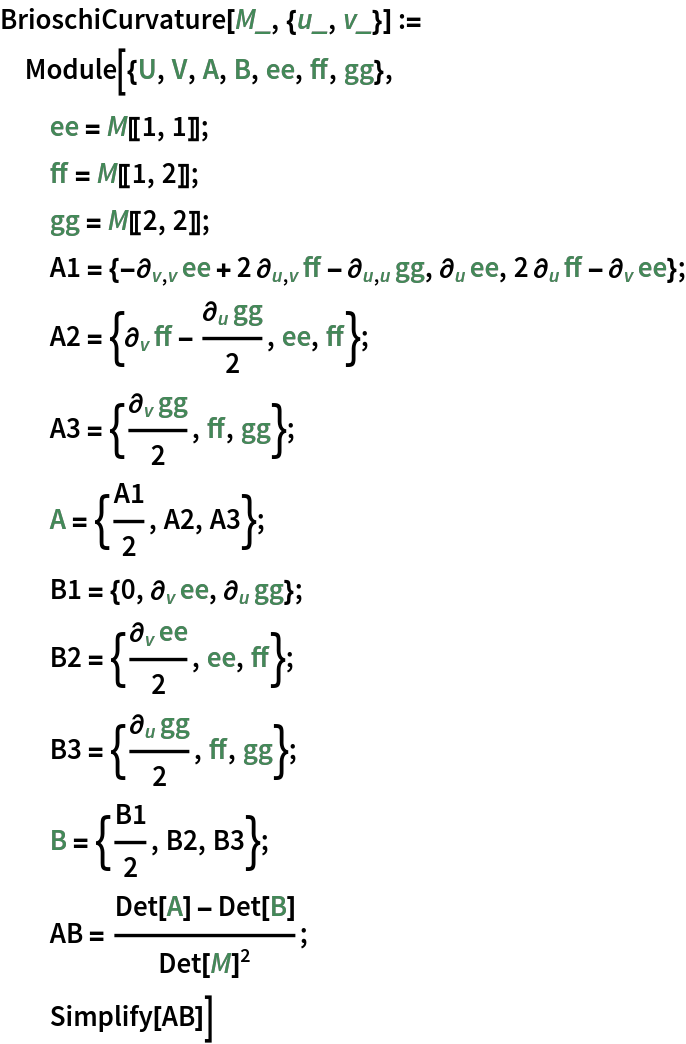Compute the Ricci scalar for the arbitrary metric:

 In:=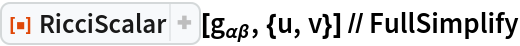Out=Prove that this coincides with twice the Gaussian curvature:

 In:=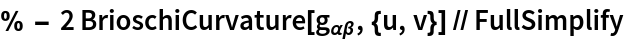Out=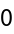Enrique Zeleny

## Version History

• 1.0.0 – 24 November 2020